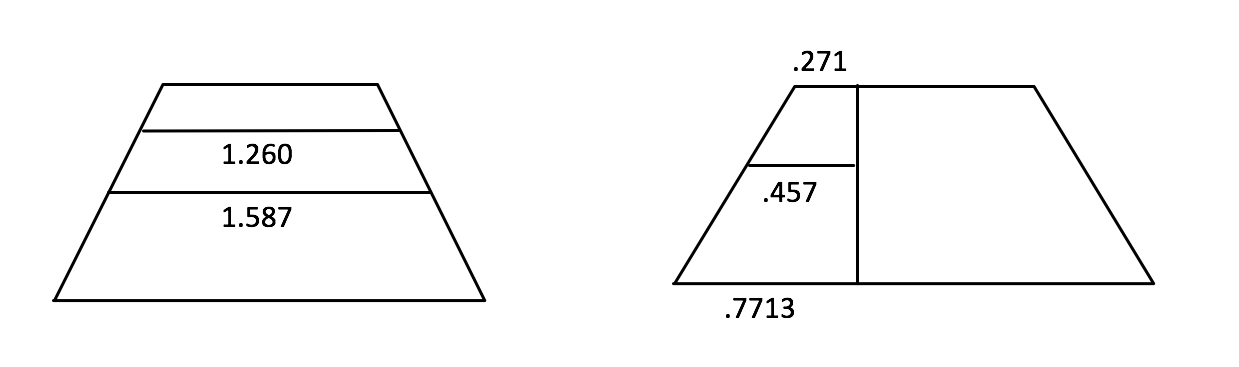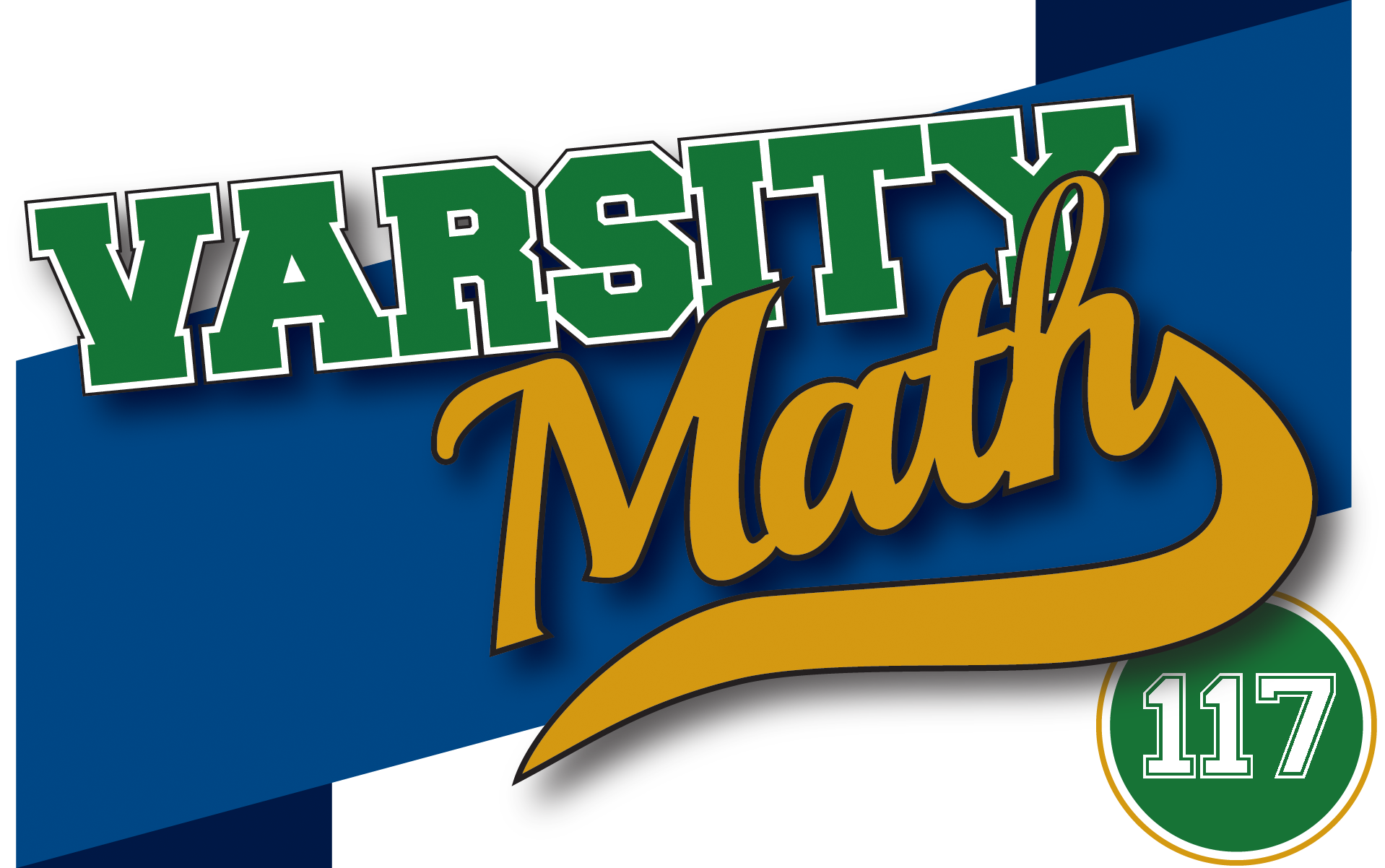## ________________

The coach decides to give the team a workout with a couple of probability problems.

## ________________### Fifty-Fifty

From a bag containing red and blue balls, two are removed at random. The chances are 50-50 that they will differ in color.

What were the possible numbers of balls initially in the bag?

### Random Toss

Teammate Joachim is tossing circular disks randomly on a floor marked out in squares. He wins if the disk lands so that it covers parts of exactly two or three squares. In the diagram, A and B are winning positions, while C and D are not. Suppose the squares have a unit side and Joachim gets to pick the diameter of the disk.

What probability of winning can Joachim achieve and what disk diameter achieves this?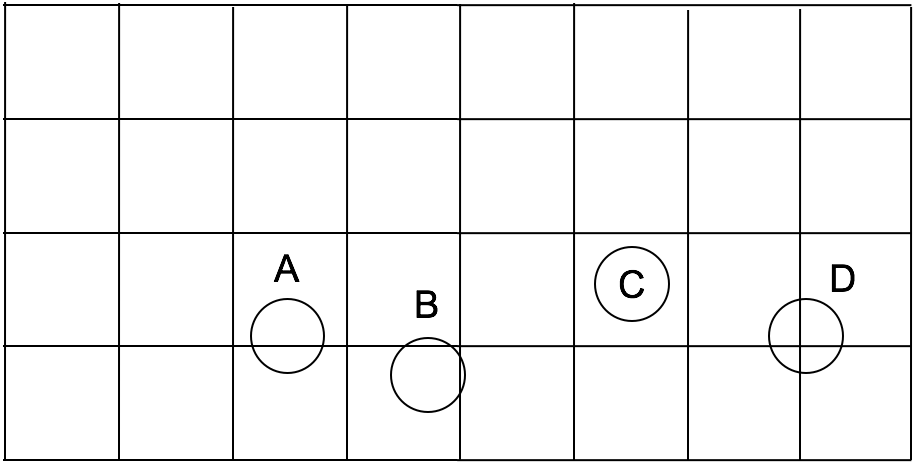## Solutions to week 116

Solutions for Divide by Three and Cutting the Domino are shown below.

Divide by Three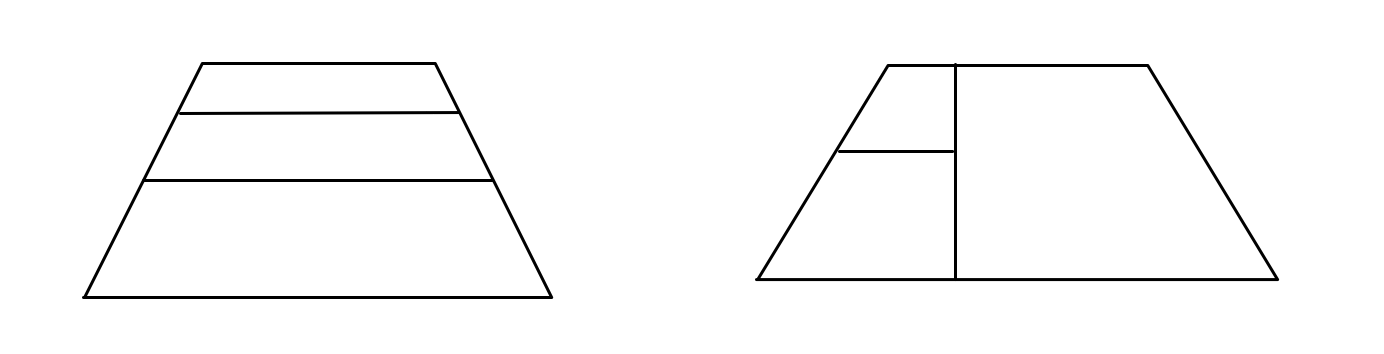Cutting the Domino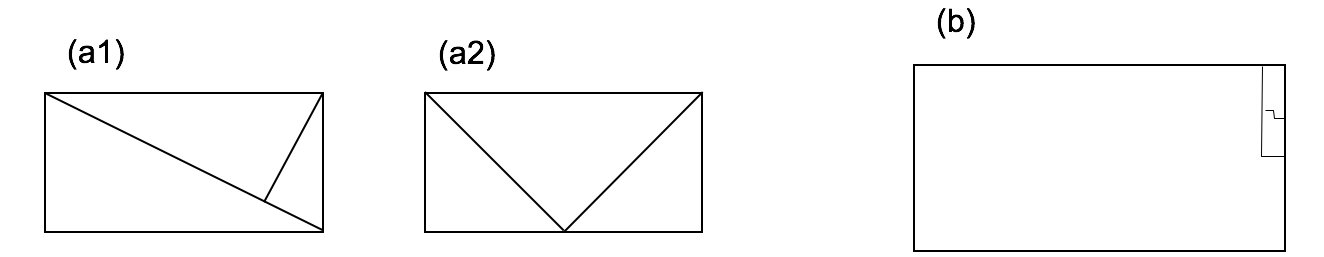Scale the half hexagon so that its three sides are of unit length. In the first solution, the smaller length is the cube root of 2 and the larger length is the cube root of 4. In the second solution, the three lengths are (9-√33)/12, (√33-3)/6, and (15-√33)/12.